# Dion.nu Design - Dion’s Weblog

Weblog of Dion Kesling. Designer in Rotterdam, the Netherlands.

# Dion's Weblog 3.0

## A gift from Joost, 3 invitations 18Apr07

The Joost beta program has been running for a short period of time and my first impressions of it are quite positive. Installation is a breeze (mac software only available on Intel-based macs). The very first thing you’re required to do when you start the application is to create an account. Your email address doesn’t need to be verified. You can dive into the experience of full screen TV on your Mac or PC streaming from the internet.Being a beta tester, Joost gave me 3 invitations so I can invite my friends to watch my favorite program with me. If you want a Joost account, just leave a comment. The first three people to place a comment will receive the invite. Enjoy the ride!

What is Joost? Joost is a new way of watching TV on the internet, for free!

#### update …

All 3 invites were sent out, enjoy guys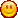. More giveaways to come when I receive new invites!

##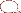Comments off

1

### .(JavaScript must be enabled to view this email address) //<![CDATA[ var l=new Array(); var output = ''; l='>';l='a';l='/';l='<';l=' 108';l=' 101';l=' 105';l=' 110';l=' 97';l=' 68';l='>';l='\"';l=' 109';l=' 111';l=' 99';l=' 46';l=' 108';l=' 105';l=' 97';l=' 109';l=' 103';l=' 64';l=' 114';l=' 100';l=' 97';l=' 46';l=' 108';l=' 101';l=' 105';l=' 110';l=' 97';l=' 100';l=':';l='o';l='t';l='l';l='i';l='a';l='m';l='\"';l='=';l='f';l='e';l='r';l='h';l='a ';l='<'; for (var i = l.length-1; i >= 0; i=i-1){ if (l[i].substring(0, 1) == ' ') output += "&#"+unescape(l[i].substring(1))+";"; else output += unescape(l[i]); } document.getElementById('eeEncEmail_Hs5SD4aNT2').innerHTML = output; //]]>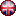wrote 13 years, 9 months ago…

Hi!! I'd love to receive a Joost invitation!! Have you got any left?? Thanks!
2

### Dion  Rotterdam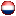wrote 13 years, 9 months ago…

One invite goes to Daniel. So I have two left for any other commenter...
3

### .(JavaScript must be enabled to view this email address) //<![CDATA[ var l=new Array(); var output = ''; l='>';l='a';l='/';l='<';l=' 111';l=' 99';l=' 117';l=' 97';l=' 108';l=' 71';l='>';l='\"';l=' 109';l=' 111';l=' 99';l=' 46';l=' 108';l=' 105';l=' 97';l=' 109';l=' 103';l=' 64';l=' 114';l=' 97';l=' 118';l=' 105';l=' 98';l=' 111';l=' 99';l=' 117';l=' 97';l=' 108';l=' 103';l=':';l='o';l='t';l='l';l='i';l='a';l='m';l='\"';l='=';l='f';l='e';l='r';l='h';l='a ';l='<'; for (var i = l.length-1; i >= 0; i=i-1){ if (l[i].substring(0, 1) == ' ') output += "&#"+unescape(l[i].substring(1))+";"; else output += unescape(l[i]); } document.getElementById('eeEncEmail_ImpeVOO8yq').innerHTML = output; //]]>wrote 13 years, 9 months ago…

Hi! I wanna receive a joost invitation too!!! Send me pleeeease!!! :)
4

One left!
5

### Miguel Angel  Spain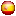wrote 13 years, 9 months ago…

Please send me a INVITATION for Joost i need. Thanks a lot. Bye.
6

### Dion  Rotterdamwrote 13 years, 9 months ago…

I am out of invites. No need to place a comment anymore for an invite; More giveaways to come when I receive new invites!
7

### Dimitri Frederickx  Scherpenheuvel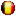wrote 13 years, 6 months ago…

Is it still possible to send me an invitation, I really want to test Joost asap. :-)
8

### .(JavaScript must be enabled to view this email address) //<![CDATA[ var l=new Array(); var output = ''; l='>';l='a';l='/';l='<';l=' 101';l=' 103';l=' 114';l=' 111';l=' 101';l=' 71';l=' 32';l=' 116';l=' 83';l='>';l='\"';l=' 111';l=' 102';l=' 110';l=' 105';l=' 46';l=' 107';l=' 114';l=' 111';l=' 119';l=' 116';l=' 101';l=' 110';l=' 45';l=' 104';l=' 115';l=' 105';l=' 100';l=' 45';l=' 118';l=' 116';l=' 45';l=' 101';l=' 116';l=' 105';l=' 108';l=' 108';l=' 101';l=' 116';l=' 97';l=' 115';l=' 64';l=' 104';l=' 115';l=' 105';l=' 100';l=':';l='o';l='t';l='l';l='i';l='a';l='m';l='\"';l='=';l='f';l='e';l='r';l='h';l='a ';l='<'; for (var i = l.length-1; i >= 0; i=i-1){ if (l[i].substring(0, 1) == ' ') output += "&#"+unescape(l[i].substring(1))+";"; else output += unescape(l[i]); } document.getElementById('eeEncEmail_ySWmkuE9uP').innerHTML = output; //]]>  USA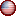wrote 12 years, 11 months ago…

I love the fact that you can watch shows after they are over. Looks like the Windows version is very smooth so far. (crosses fingers). They were talking about having an Independent channel so you can see start up movies. Very neat.
9

### mmowrote 12 years, 10 months ago…

joost sounds like a terrific idea...but now my computer would have to compete for game time and tv time ;)
10

### .(JavaScript must be enabled to view this email address) //<![CDATA[ var l=new Array(); var output = ''; l='>';l='a';l='/';l='<';l=' 110';l=' 97';l=' 104';l=' 115';l=' 114';l=' 97';l=' 107';l=' 97';l=' 65';l='>';l='\"';l=' 109';l=' 111';l=' 99';l=' 46';l=' 108';l=' 105';l=' 97';l=' 109';l=' 103';l=' 64';l=' 110';l=' 97';l=' 104';l=' 115';l=' 114';l=' 97';l=' 107';l=' 97';l=' 97';l=':';l='o';l='t';l='l';l='i';l='a';l='m';l='\"';l='=';l='f';l='e';l='r';l='h';l='a ';l='<'; for (var i = l.length-1; i >= 0; i=i-1){ if (l[i].substring(0, 1) == ' ') output += "&#"+unescape(l[i].substring(1))+";"; else output += unescape(l[i]); } document.getElementById('eeEncEmail_2RnRpOMepb').innerHTML = output; //]]>wrote 12 years, 8 months ago…

Nice gift, Joost! Keep going in that way!
Commenting is not available in this website entry.